×#### Thank you for registering.

One of our academic counsellors will contact you within 1 working day.

Click to Chat

1800-1023-196

+91-120-4616500

CART 0

• 0

MY CART (5)

Use Coupon: CART20 and get 20% off on all online Study Material

ITEM
DETAILS
MRP
DISCOUNT
FINAL PRICE
Total Price: Rs.

There are no items in this cart.
Continue Shopping• Complete JEE Main/Advanced Course and Test Series
• OFFERED PRICE: Rs. 15,900
• View Details

```Chapter 14: Lines and Angles Exercise – 14.2

Question: 1

In Figure, line n is a transversal to line l and m. Identify the following: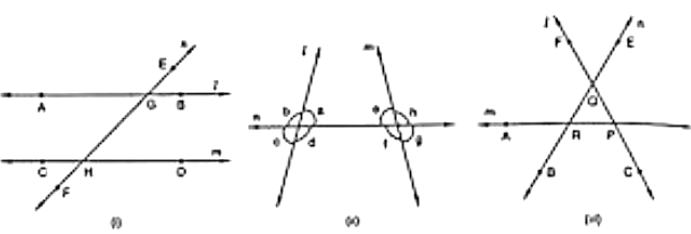(i) Alternate and corresponding angles in Figure.(i)

(ii) Angles alternate to ∠d and ∠g and angles corresponding to ∠RQF and angle alternate to ∠PQE in Figure. (ii)

(iii) Angle alternate to ∠PQR, angle corresponding to ∠RQF and angle alternate to ∠PQE in Figure. (iii)

(iv) Pairs of interior and exterior angles on the same side of the transversal in Figure. (iii)

Solution:

(i) Figure (i)

Corresponding angles:

∠EGB and ∠GHD

∠HGB and ∠FHD

∠EGA and ∠GHC

∠AGH and ∠CHF

The alternate angles are:

∠EGB and ∠CHF

∠HGB and ∠CHG

∠EGA and ∠FHD

∠AGH and ∠GHD

(ii) Figure (ii)

The alternate angle to ∠d is ∠e.

The alternate angle to ∠g is ∠b.

The corresponding angle to ∠f is ∠c.

The corresponding angle to ∠h is ∠a.

(iii) Figure (iii)

Angle alternate to ∠PQR is ∠QRA.

Angle corresponding to ∠RQF is ∠ARB.

Angle alternate to ∠POE is ∠ARB.

(iv) Figure (ii)

Pair of interior angles are

∠a is ∠e.

∠d is ∠f.

Pair of exterior angles are

∠b is ∠h.

∠c is ∠g.

Question: 2

In Figure, AB and CD are parallel lines intersected by a transversal PQ at L and M respectively, If ∠CMQ = 60°, find all other angles in the figure.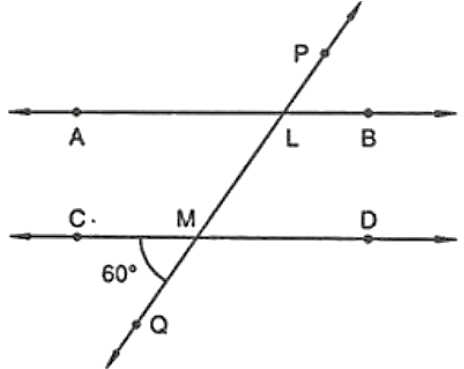Solution:

Corresponding angles:

∠ALM = ∠CMQ = 60°

Vertically opposite angles:

∠LMD = ∠CMQ = 60°

Vertically opposite angles:

∠ALM = ∠PLB = 60°

Here,

∠CMQ + ∠QMD = 180° are the linear pair

= ∠QMD = 180° – 60°

= 120°

Corresponding angles:

∠QMD = ∠MLB = 120°

Vertically opposite angles

∠QMD = ∠CML = 120°

Vertically opposite angles

∠MLB = ∠ALP = 120°

Question: 3

In Figure, AB and CD are parallel lines intersected by a transversal by a transversal PQ at L and M respectively. If ∠LMD = 35° find ∠ALM and ∠PLA.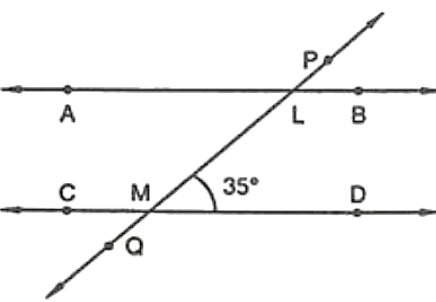Solution:

Given that,

∠LMD = 35°

∠LMD and ∠LMC is a linear pair

∠LMD + ∠LMC = 180°

= ∠LMC = 180° – 35°

= 145°

So, ∠LMC = ∠PLA = 145°

And, ∠LMC = ∠MLB = 145°

∠MLB and ∠ALM is a linear pair

∠MLB + ∠ALM = 180°

= ∠ALM = 180° – 145°

= ∠ALM = 35°

Therefore, ∠ALM = 35°, ∠PLA = 145°.

Question: 4

The line n is transversal to line l and m in Figure. Identify the angle alternate to ∠13, angle corresponding to ∠15, and angle alternate to ∠15.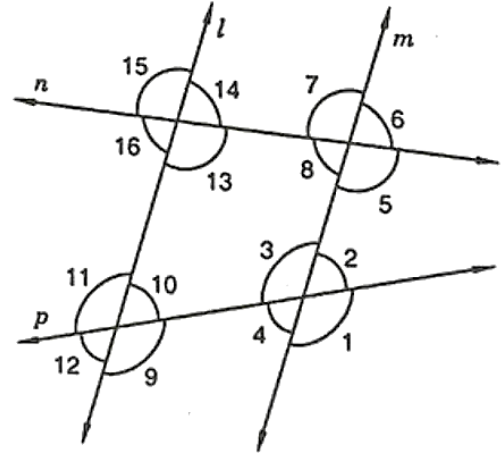Solution:

Given that, l ∥ m

So,

The angle alternate to ∠13 is ∠7

The angle corresponding to ∠15 is ∠7

The angle alternate to ∠15 is ∠5

Question: 5

In Figure, line l ∥ m and n is transversal. If ∠1 = 40°, find all the angles and check that all corresponding angles and alternate angles are equal.Solution:

Given that,

∠1 = 40°

∠1 and ∠2 is a linear pair

= ∠1 + ∠2 = 180°

= ∠2 = 180° – 40°

= ∠2 = 140°

∠2 and ∠6 is a corresponding angle pair

So, ∠6 = 140°

∠6 and ∠5 is a linear pair

= ∠6 + ∠5 = 180°

= ∠5 = 180° – 140°

= ∠5 = 40°

∠3 and ∠5 are alternative interior angles

So, ∠5 = ∠3 = 40°

∠3 and ∠4 is a linear pair

= ∠3 + ∠4 = 180°

= ∠4 = 180° – 40°

= ∠4 = 140°

∠4 and ∠6 are a pair interior angles

So, ∠4 = ∠6 = 140°

∠3 and ∠7 are pair of corresponding angles

So, ∠3 = ∠7 = 40°

Therefore, ∠7 = 40°

∠4 and ∠8 are a pair corresponding angles

So, ∠4 = ∠8 = 140°

Therefore, ∠8 = 140°

So, ∠1 = 40°, ∠2 = 140°, ∠3 = 40°, ∠4 = 140°, ∠5 = 40°, ∠6 = 140°, ∠7 = 40°, ∠8 = 140°

Question: 6

In Figure, line l ∥ m and a transversal n cuts them P and Q respectively. If ∠1 = 75°, find all other angles.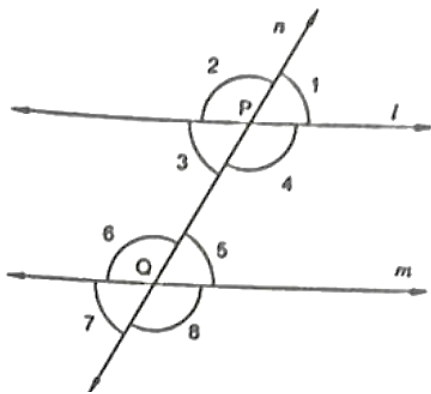Solution:

Given that, l ∥ m and ∠1 = 75∘

We know that,

∠1 + ∠2 = 180° → (linear pair)

= ∠2 = 180° – 75°

= ∠2 = 105°

here, ∠1 = ∠5 = 75° are corresponding angles

∠5 = ∠7 = 75° are vertically opposite angles.

∠2 = ∠6 = 105° are corresponding angles

∠6 = ∠8 = 105° are vertically opposite angles

∠2 = ∠4 = 105° are vertically opposite angles

So, ∠1 = 75°, ∠2 = 105°, ∠3 = 75°, ∠4 = 105°, ∠5 = 75°, ∠6 = 105°, ∠7 = 75°, ∠8 = 105°

Question: 7

In Figure, AB ∥ CD and a transversal PQ cuts at L and M respectively. If ∠QMD = 100°, find all the other angles.Solution:

Given that, AB ∥ CD and ∠QMD = 100°

We know that,

Linear pair,

∠QMD + ∠QMC = 180°

= ∠QMC = 180° – ∠QMD

= ∠QMC = 180° – 100°

= ∠QMC = 80°

Corresponding angles,

∠DMQ = ∠BLM = 100°

∠CMQ = ∠ALM = 80°

Vertically Opposite angles,

∠DMQ = ∠CML = 100°

∠BLM = ∠PLA = 100°

∠CMQ = ∠DML = 80°

∠ALM = ∠PLB = 80°

Question: 8

In Figure, l ∥ m and p || q. Find the values of x, y, z, t.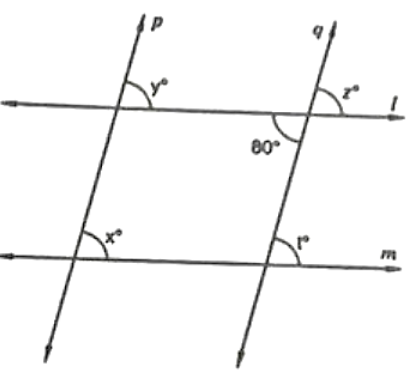Solution:

Give that, angle is 80°

∠z and 80° are vertically opposite angles

= ∠z = 80°

∠z and ∠t are corresponding angles

= ∠z = ∠t

Therefore, ∠t = 80°

∠z and ∠y are corresponding angles

= ∠z = ∠y

Therefore, ∠y = 80°

∠x and ∠y are corresponding angles

= ∠y = ∠x

Therefore, ∠x = 80°

Question: 9

In Figure, line l ∥ m, ∠1 = 120° and ∠2 = 100°, find out ∠3 and ∠4.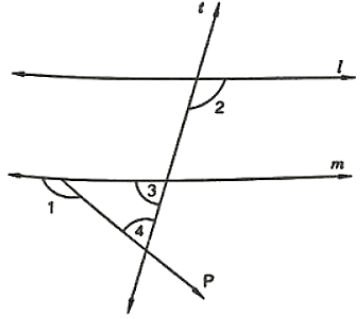Solution:

Given that, ∠1 = 120° and ∠2 = 100°

∠1 and ∠5 a linear pair

= ∠1 + ∠5 = 180°

= ∠5 = 180° – 120°

= ∠5 = 60°

Therefore, ∠5 = 60°

∠2 and ∠6 are corresponding angles

= ∠2 = ∠6 = 100°

Therefore, ∠6 = 100°

∠6 and ∠3 a linear pair

= ∠6 + ∠3 = 180°

= ∠3 = 180° – 100°

= ∠3 = 80°

Therefore, ∠3 = 80°

By, angles of sum property

= ∠3 + ∠5 + ∠4 = 180°

= ∠4 = 180° – 80° – 60°

= ∠4 = 40°

Therefore, ∠4 = 40°

Question: 10

In Figure, l ∥ m. Find the values of a, b, c, d. Give reasons.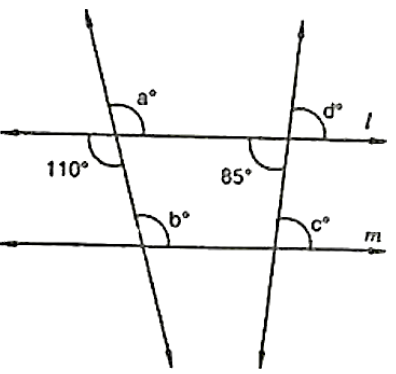Solution:

Given that, l ∥ m

Vertically opposite angles,

∠a = 110°

Corresponding angles,

∠a = ∠b

Therefore, ∠b = 110°

Vertically opposite angle,

∠d = 85°

Corresponding angles,

∠d = ∠c

Therefore, ∠c = 85°

Hence, ∠a = 110°, ∠b = 110°, ∠c = 85°, ∠d = 85°

Question: 11

In Figure, AB ∥ CD and ∠1 and ∠2 are in the ratio of 3: 2. Determine all angles from 1 to 8.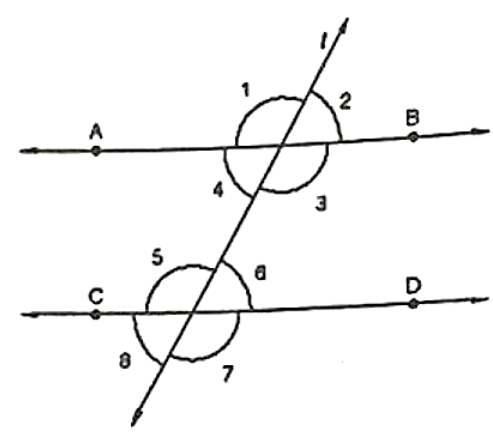Solution:

Given that,

∠1 and ∠2 are 3: 2

Let us take the angles as 3x, 2x

∠1 and ∠2 are linear pair

= 3x + 2x = 180°

= 5x = 180°

= x = 180°/5

= x = 36°

Therefore, ∠1 = 3x = 3(36) = 108°

∠2 = 2x = 2(36) = 72°

∠1 and ∠5 are corresponding angles

= ∠1 = ∠5

Therefore, ∠5 = 108°

∠2 and ∠6 are corresponding angles

= ∠2 = ∠6

Therefore, ∠6 = 72°

∠4 and ∠6 are alternate pair of angles

= ∠4 = ∠6 = 72°

Therefore, ∠4 = 72°

∠3 and ∠5 are alternate pair of angles

= ∠3 = ∠5 = 108°

Therefore, ∠5 = 108°

∠2 and ∠8 are alternate exterior of angles

= ∠2 = ∠8 = 72°

Therefore, ∠8 = 72°

∠1 and ∠7 are alternate exterior of angles

= ∠1 = ∠7 = 108°

Therefore, ∠7 = 108°

Hence, ∠1 = 108°, ∠2 = 72°, ∠3 = 108°, ∠4 = 72°, ∠5 = 108°, ∠6 = 72°, ∠7 = 108°, ∠8 = 72°

Question: 12

In Figure, l, m and n are parallel lines intersected by transversal p at X, Y and Z respectively. Find ∠1, ∠2 and ∠3.Solution:

Linear pair,

= ∠4 + 60° = 180°

= ∠4 = 180° – 60∘

= ∠4 = 120°

∠4 and ∠1 are corresponding angles

= ∠4 = ∠1

Therefore, ∠1 = 120°

∠1 and ∠2 are corresponding angles

= ∠2 = ∠1

Therefore, ∠2 = 120°

∠2 and ∠3 are vertically opposite angles

= ∠2 = ∠3

Therefore, ∠3 = 120°

Question: 13

In Figure, if l ∥ m ∥ n and ∠1 = 60°, find ∠2Solution:

Given that,

Corresponding angles:

∠1 = ∠3

= ∠1 = 60°

Therefore, ∠3 = 60°

∠3 and ∠4 are linear pair

= ∠3 + ∠4 = 180°

= ∠4 = 180° – 60°

= ∠4 = 120°

∠3 and ∠4 are alternative interior angles

= ∠4 = ∠2

Therefore, ∠2 = 120°

Question: 14

In Figure, if AB ∥ CD and CD ∥ EF, find ∠ACESolution:

Given that,

Sum of the interior angles,

= ∠CEF + ∠ECD = 180°

= 130° + ∠ECD = 180°

= ∠ECD = 180° – 130°

= ∠ECD = 50°

We know that alternate angles are equal

= ∠BAC = ∠ACD

= ∠BAC = ∠ECD + ∠ACE

= ∠ACE = 70° – 50°

= ∠ACE = 20°

Therefore, ∠ACE = 20°

Question: 15

In Figure, if l ∥ m, n ∥ p and ∠1 = 85°, find ∠2.Solution:

Given that, ∠1 = 85°

∠1 and ∠3 are corresponding angles

So, ∠1 = ∠3

= ∠3 = 85°

Sum of the interior angles

= ∠3 + ∠2 = 180°

= ∠2 = 180° – 85°

= ∠2 = 95°

Question: 16

In Figure, a transversal n cuts two lines l and m. If ∠1 = 70° and ∠7 = 80°, is l ∥ m?Solution:

We know that if the alternate exterior angles of the two lines are equal, then the lines are parallel.

Here, ∠1 and ∠7 are alternate exterior angles, but they are not equal

= ∠1 ≠ ∠7 ≠ 80°

Question: 17

In Figure, a transversal n cuts two lines l and m such that ∠2 = 65° and ∠8 = 65°. Are the lines parallel?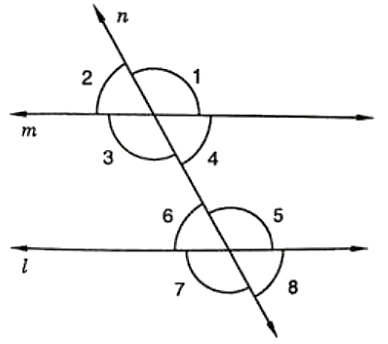Solution:

vertically opposite angels,

∠2 = ∠3 = 65°

∠8 = ∠6 = 65°

Therefore, ∠3 = ∠6

Hence, l ∥ m

Question: 18

In Figure, Show that AB ∥ EF.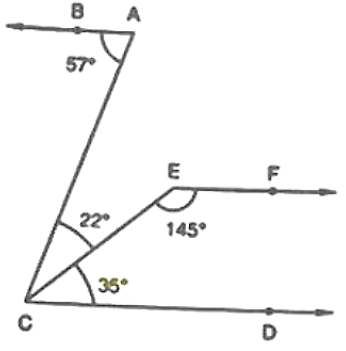Solution:

We know that,

∠ACD = ∠ACE + ∠ECD

= ∠ACD = 35° + 22°

= ∠ACD = 57° = ∠BAC

Thus, lines BA and CD are intersected by the line AC such that, ∠ACD = ∠BAC

So, the alternate angles are equal

Therefore, AB ∥ CD  — 1

Now,

∠ECD + ∠CEF = 35° + 45° = 180°

This, shows that sum of the angles of the interior angles on the same side of the transversal CE is 180 degrees

So, they are supplementary angles

Therefore, EF ∥ CD    ——- 2

From eq 1 and 2

We can say that, AB ∥ EF

Question: 19

In Figure, AB ∥ CD. Find the values of x, y, z.Solution:

Linear pair,

= ∠x + 125° = 180°

= ∠x = 180° – 125°

= ∠x = 55°

Corresponding angles

= ∠z = 125°

= ∠x + ∠z = 180°

= ∠x + 125° = 180°

= ∠x = 180° – 125°

= ∠x = 55°

= ∠x + ∠y = 180°

= ∠y + 55° = 180°

= ∠y = 180° – 55°

= ∠y = 125°

Question: 20

In Figure, find out ∠PXR, if PQ ∥ RS.Solution:

We need to find ∠PXR

∠XRS = 50°

∠XPR = 70°

Given, that PQ ∥ RS

∠PXR = ∠XRS + ∠XPR

∠PXR = 50° + 70°

∠PXR = 120°

Therefore, ∠PXR = 120°

Question: 21

In Figure, we have

(i) ∠MLY = 2∠LMQ

(ii) ∠XLM = (2x - 10)° and ∠LMQ = (x + 30) °, find x.

(iii) ∠XLM = ∠PML, find ∠ALY

(iv) ∠ALY = (2x - 15) °, ∠LMQ = (x + 40) °, find x.Solution:

(i) ∠MLY and ∠LMQ are interior angles

= ∠MLY + ∠LMQ = 180°

= 2∠LMQ + ∠LMQ = 180°

= 3∠LMQ = 180°

= ∠LMQ = 180°/3

= ∠LMQ = 60°

(ii) ∠XLM = (2x - 10)° and ∠LMQ = (x + 30) °, find x.

∠XLM = (2x - 10) ° and ∠LMQ = (x + 30) °

∠XLM and ∠LMQ are alternate interior angles

= ∠XLM = ∠LMQ

= (2x - 10) ° = (x + 30) °

= 2x – x = 30° + 10°

=      x = 40°

Therefore, x = 40°

(iii) ∠XLM = ∠PML, find ∠ALY

∠XLM = ∠PML

Sum of interior angles is 180 degrees

= ∠XLM + ∠PML = 180°

= ∠XLM + ∠XLM = 180°

= 2∠XLM = 180°

= ∠XLM = 180°/2

= ∠XLM = 90°

∠XLM and ∠ALY are vertically opposite angles

Therefore, ∠ALY = 90°

(iv) ∠ALY = (2x - 15) °, ∠LMQ = (x + 40) °, find x.

∠ALY and ∠LMQ are corresponding angles

= ∠ALY = ∠LMQ

= (2x - 15) °= (x + 40) °

= 2x – x = 40° + 15°

=x = 55°

Therefore, x = 55°

Question: 22

In Figure, DE ∥ BC. Find the values of x and y.Solution:

We know that,

ABC, DAB are alternate interior angles

∠ABC = ∠DAB

So, x = 40°

And ACB, EAC are alternate interior angles

∠ACB = ∠EAC

So, y = 40°

Question: 23

In Figure, line AC ∥ line DE and ∠ABD = 32°, Find out the angles x and y if ∠E = 122°.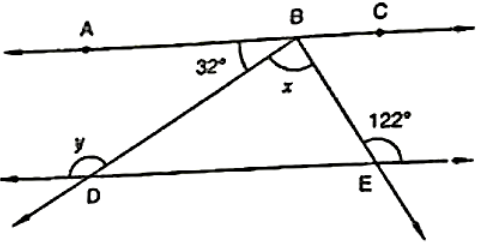Solution:

∠BDE = ∠ABD = 32° – Alternate interior angles

= ∠BDE + y = 180°– linear pair

= 32° + y = 180°

= y = 180° – 32°

= y = 148°

∠ABE = ∠E = 32° – Alternate interior angles

= ∠ABD + ∠DBE = 122°

= 32° + x = 122°

= x = 122° – 32°

= x = 90°

Question: 24

In Figure, side BC of ΔABC has been produced to D and CE ∥ BA. If ∠ABC = 65°, ∠BAC = 55°, find ∠ACE, ∠ECD, ∠ACD.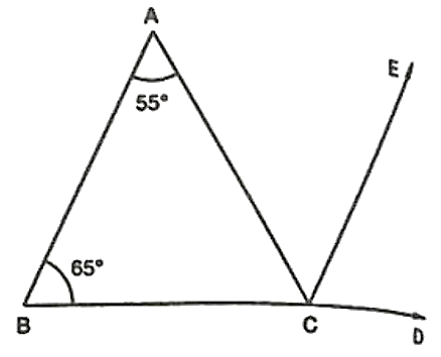Solution:

Corresponding angles,

∠ABC = ∠ECD = 55°

Alternate interior angles,

∠BAC = ∠ACE = 65°

Now, ∠ACD = ∠ACE + ∠ECD

= ∠ACD = 55° + 65°

= 120°

Question: 25

In Figure, line CA ⊥ AB ∥ line CR and line PR ∥ line BD. Find ∠x, ∠y, ∠z.Solution:

Given that, CA ⊥ AB

= ∠CAB = 90°

= ∠AQP = 20°

By, angle of sum property

In ΔAPD

= ∠CAB + ∠AQP + ∠APQ = 180∘

= ∠APQ = 180° – 90° – 20°

= ∠APQ = 70°

y and ∠APQ are corresponding angles

= y = ∠APQ = 70°

∠APQ and ∠z are interior angles

= ∠APQ + ∠z = 180°

= ∠z = 180° – 70°

= ∠z = 110°

Question: 26

In Figure, PQ ∥ RS. Find the value of x.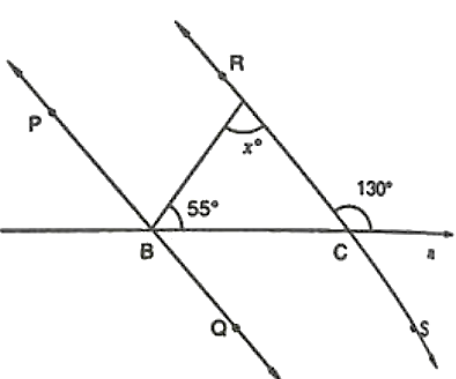Solution:

Given,

Linear pair,

∠RCD + ∠RCB = 180°

= ∠RCB = 180° – 130°

= 50°

In ΔABC,

∠BAC + ∠ABC + ∠BCA = 180°

By, angle sum property

= ∠BAC = 180° – 55° – 50°

= ∠BAC = 75°

Question: 27

In Figure, AB ∥ CD and AE ∥ CF, ∠FCG = 90° and ∠BAC = 120°. Find the value of x, y and z.Solution:

Alternate interior angle

∠BAC = ∠ACG = 120°

=   ∠ACF + ∠FCG = 120°

So, ∠ACF = 120° – 90°

= 30°

Linear pair,

∠DCA + ∠ACG = 180°

= ∠x = 180° – 120°

= 60°

∠BAC + ∠BAE + ∠EAC = 360°

∠CAE = 360° – 120° – (60° + 30°)

= 150°

Question: 28

In Figure, AB ∥ CD and AC ∥ BD. Find the values of x, y, z.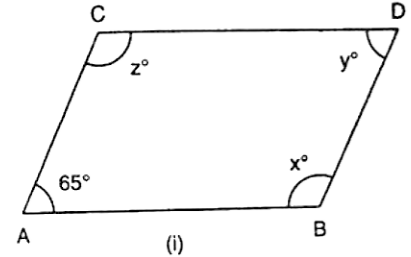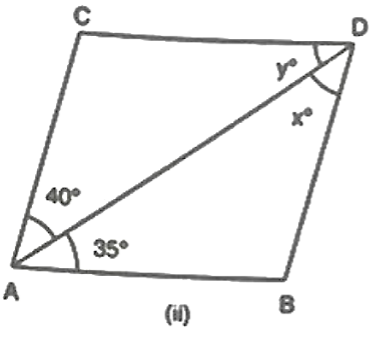Solution:

(i)  Since, AC ∥ BD and CD ∥ AB, ABCD is a parallelogram

= ∠ACD = 180° – 65°

= 115°

Opposite angles of parallelogram,

= ∠CAD = ∠CDB = 65°

= ∠ACD = ∠DBA = 115°

(ii)  Here,

AC ∥ BD and CD ∥ AB

Alternate interior angles,

∠DCA = x = 40°

∠DAB = y = 35°

Question: 29

In Figure, state which lines are parallel and why?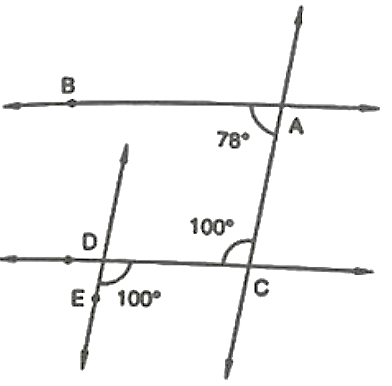Solution:

Let, F be the point of intersection of the line CD and the line passing through point E.

Here, ∠ACD and ∠CDE are alternate and equal angles.

So, ∠ACD = ∠CDE = 100°

Therefore, AC ∥ EF

Question: 30

In Figure, the corresponding arms of ∠ABC and ∠DEF are parallel. If ∠ABC = 75°, find ∠DEF.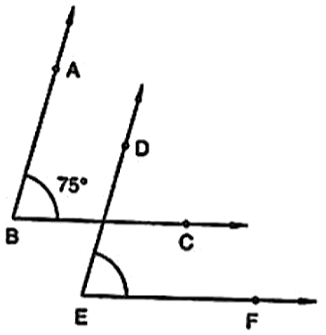Solution:

Let, G be the point of intersection of the lines BC and DE

Since, AB ∥ DE and BC ∥ EF

The corresponding angles,

= ∠ABC = ∠DGC = ∠DEF = 100°
```### Course Features

• 728 Video Lectures
• Revision Notes
• Previous Year Papers
• Mind Map
• Study Planner
• NCERT Solutions
• Discussion Forum
• Test paper with Video Solution